## Common Core Nuggets :: Conics

This collection contains apps that will help teachers implement Common Core Geometry Standard G-GPE 1, 3 for High School Mathematics.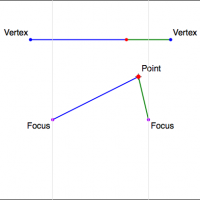#### Ellipse Definition

This app allows you to experiment with the definition of an ellipse as the set of points in which the sum of the distances from two fixed points is a constant. (G-GPE 3)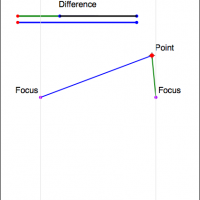#### Hyperbola Definition

This app allows you to experiment with the definition of a hyperbola as the set of points in which the difference of the distances from two fixed points is a constant. (G-GPE 3)#### Alternate Ellipse Construction

This app allows you to experiment with an alternate construction of an ellipse. The tracing of the perpendicular bisector of the segment between a point on a circle and a point in the circle create an elliptical locus. (G-GPE 3)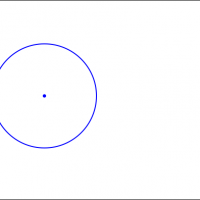#### Alternate Hyperbola Construction

This app allows you to experiment with an alternate construction of a hyperbola. The tracing of the perpendicular bisector of the segment between a point on a circle and a point outside the circle create a hyperbolic locus. (G-GPE 3)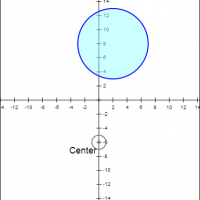#### Circle Equation

This app allows you to practice writing the equation of a circle given a graph. (G-GPE 1)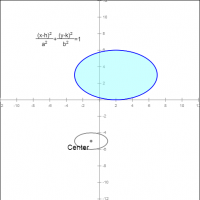#### Ellipse Equations

This app allows you to practice writing the equation of an ellipse (horizontal and vertical) given a graph. (G-GPE 3)#### Hyperbola Equations

This app allows you to practice writing the equation of a hyperbola (horizontal and vertical) given a graph.

Apps created with Geometry Expressions